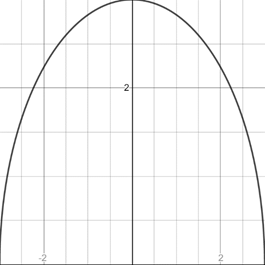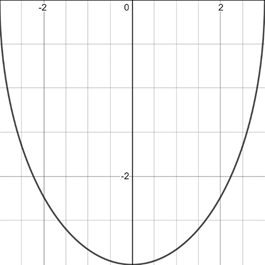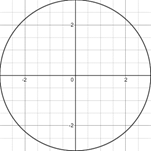# To graph : The circle x 2 + y 2 = 9 by solve for y .### Precalculus: Mathematics for Calcu...

6th Edition
Stewart + 5 others
Publisher: Cengage Learning
ISBN: 9780840068071### Precalculus: Mathematics for Calcu...

6th Edition
Stewart + 5 others
Publisher: Cengage Learning
ISBN: 9780840068071

#### Solutions

Chapter 1.9, Problem 27E
To determine

## To graph: The circle x2+y2=9 by solve for y .

Expert Solution

### Explanation of Solution

Given information:

The equation of circle x2+y2=9 .

Graph:

The graph of circle x2+y2=9 by solving for y .

Subtract x2 from the equation of circle,

x2x2+y2=9x2y2=9x2

Take under root on both side of the equation.

y=±9x2

From the two equations graph of circle is described:

y=+9x2,y=9x2

The graph of the equation y=+9x2 represents upper half of the circle because y is always greater than or equal to 0.

9x209x209x2±3x

So, the values of x is less than or equal to ±3 .

Thus, the graph appropriate in the [3,3]×[0,3] viewing rectangle

The graph of the equation can be sketched using the table,

 x y 0 3 2 5 3 0 1 8

The graph of equation is provided below,The graph of the equation y=9x2 represents lower half of the circle because y is always less than or equal to 0.

9x209x209x2±3x

So, the values of x is greater than or equal to ±3 .

Thus, the graph appropriate in the [3,3]×[-3,0] viewing rectangle

The graph of the equation y=9x2 can be sketched using the table,

 x y 0 −3 2 −5 3 0 1 −8

The graph of equation is provided below,The graph of both equations in same figure is provided below,

The graph appropriate in the [3,3]×[-3,3] viewing rectangleInterpretation:

The graph of an equation in a viewing screen is a viewing rectangle.

The x -values to range from a minimum value of xmin =a to a maximum value of xmax =b

The y -values to range from minimum value of ymin =c to a maximum value of ymax =d

Then, the display portion of the graph lies in the rectangle [a,b]×[c,d]={(x,y)|axb,cyd}

The graph of y=+9x2 appropriate in the [3,3]×[0,3] viewing rectangle and the graph of this equation lies on the above part or the graph make a half circle.

The graph of y=9x2 appropriate in the [3,3]×[-3,0] viewing rectangle and the graph of this equation lies on the lower part or the graph make a half circle.

The graph of x2+y2=9 appropriate in the [3,3]×[-3,3] viewing rectangle and the graph make a circle.

### Have a homework question?

Subscribe to bartleby learn! Ask subject matter experts 30 homework questions each month. Plus, you’ll have access to millions of step-by-step textbook answers!